The Principle of Relativity: A Collection of Original Memoirs on the Special and General Theory of Relativity

Contents:
Author: Albert Einstein  | Date: 1905

W. Perrett and G.B. Jeffery Physics

# Does the Inertia of a Body Depend Upon Its Energy-Content?

A. EINSTEIN

Translated from "Ist die Trägheit eines Körpers yon seinem Energiegehalt abhängig?" Annalen der Physik, 17, 1905.

# Does the Inertia of a Body Depend Upon Its Energy-Content?

BY A. EINSTEIN

THE results of the previous investigation lead to a very interesting conclusion, which is here to be deduced.

I based that investigation on the Maxwell-Hertz equations for empty space, together with the Maxwellian expression for the electromagnetic energy of space, and in addition the principle that:—

The laws by which the states of physical systems alter are independent of the alternative, to which of two systems of coordinates, in uniform motion of parallel translation relatively to each other, these alterations of state are referred (principle of relativity).

With these principles* as my basis I deduced inter alia the following result (§ 8):—

Let a system of plane waves of light, referred to the system of co-ordinates (x, y, z), possess the energy l; let the direction of the ray (the wave-normal) make an angle ø with the axis of x of the system. If we introduce a new system of co-ordinates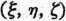moving m uniform parallel translation with respect to the system (x, y, z), and having its origin of co-ordinates in motion along the axis of x with the velocity v, then this quantity of light—measured in the system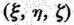—possesses the energy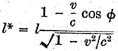where e denotes the velocity of light. We shall make use of this result in what follows.

Let there be a stationary body in the system (x, y, z), and let its energy—referred to the system (x, y, z)—be E0. Let the energy of the body relative to the system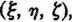moving as above with the velocity v, be H0.

Let this body send out, in a direction making an angle ø with the axis of x, plane waves of light, of energy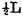measured relatively to (x, y, z), and simultaneously an equal quantity of light in the opposite direction. Meanwhile the body remains at rest with respect to the system (x, y, z). The principle of energy must apply to this process, and in fact (by the principle of relativity) with respect to both systems of co-ordinates. If we call the energy of the body after the emission of light E1 or Hi respectively, measured relatively to the system (x, y, z) or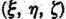respectively, then by employing the relation given above we obtain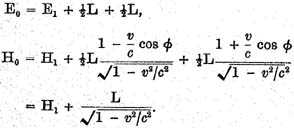By subtraction we obtain from these equations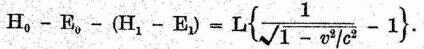The two differences of the form H - E occurring in this expression have simple physical significations. H and E are energy values of the same body referred to two systems of co-ordinates which are in motion relatively to each other, the body being at rest in one of the two systems (system (x, y, z)). Thus it is clear that the difference H - E can differ from the kinetic energy K of the body, with respect to the other system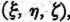only by an additive constant C, which depends on the choice of the arbitrary additive constants of the energies H and E. Thus we may place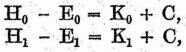since C does not change during the emission of light. So we have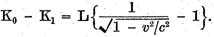The kinetic energy of the body with respect to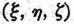diminishes as a result of the emission of light, and the amount of diminution is independent of the properties of the body. Moreover, the difference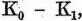like the kinetic energy of the electron (§ 10), depends on the velocity.

Neglecting magnitudes of fourth and higher orders we may place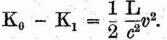From this equation it directly follows that:—

If a body gives off the energy L in the form of radiation, its mass diminishes by L/c2. The fact that the energy withdrawn from the body becomes energy of radiation evidently makes no difference, so that we are led to the more general conclusion that

The mass of a body is a measure of its energy-content; if the energy changes by L, the mass changes in the same sense by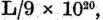the energy being measured in ergs, and the mass m grammes.

It is not impossible that with bodies whose energy-content is variable to a high degree (e.g. with radium salts) the theory may be successfully put to the test.

If the theory corresponds to the facts, radiation conveys inertia between the emitting and absorbing bodies.

* The principle of the constancy of the velocity of light is of course contained in Maxwell’s equations.

Contents:

### Related Resources

Albert Einstein
Classics of Non-fiction

Title: The Principle of Relativity: A Collection of Original Memoirs on the Special and General Theory of Relativity

Select an option:

*Note: A download may not start for up to 60 seconds.

## Email Options

Title: The Principle of Relativity: A Collection of Original Memoirs on the Special and General Theory of Relativity

Select an option: